# 2.1   Elementary Sorts

In this section, we shall study two elementary sorting methods (selection sort and insertion sort) and a variation of one of them (shellsort).

## Rules of the game.

Our primary concern is algorithms for rearranging arrays of items where each item contains a key. The objective is to rearrange the items such that their keys are in ascending order. In Java, the abstract notion of a key is captured in a built-in mechanism—the Comparable interface. With but a few exceptions, our sort code refers to the data only through two operations: the method less() that compares objects and the method exch() that exchanges them.
 private static boolean less(Comparable v, Comparable w) { return (v.compareTo(w) < 0); } private static void exch(Comparable[] a, int i, int j) { Comparable swap = a[i]; a[i] = a[j]; a[j] = swap; } 

• Sorting cost model. When studying sorting algorithms, we count compares and exchanges. For algorithms that do not use exchanges, we count array accesses.

• Extra memory. The sorting algorithms we consider divide into two basic types: those that sort in place (no extra memory except perhaps for a small function-call stack or a constant number of instance variables), and those that need enough extra memory to hold another copy of the array to be sorted.

• Types of data. Our sort code is effective for any type of data that implements Java's Comparable interface. This means that there is a method compareTo() for which v.compareTo(w) returns an integer that is negative, zero, or positive when v < w, v = w, or v > w, respectively. The method must implement a total order:
• Reflexive: for all v, v = v.
• Antisymmetric: for all v and w, if (v < w) then (w > v); and if (v = w) then (w = v).
• Transitive: for all v, w, and x, if (v ≤ w) and (w ≤ x), then v ≤ x.

In addition, v.compareTo(w) must throw an exception if v and w are of incompatible types or if either is null.

Date.java illustrates how to implement the Comparable interface for a user-defined type.

## Selection sort.

One of the simplest sorting algorithms works as follows: First, find the smallest item in the array, and exchange it with the first entry. Then, find the next smallest item and exchange it with the second entry. Continue in this way until the entire array is sorted. This method is called selection sort because it works by repeatedly selecting the smallest remaining item. Selection.java is an implementation of this method.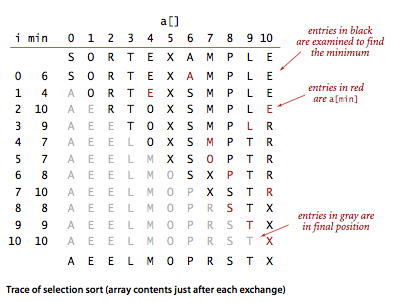### Proposition.

Selection sort uses ~n2/2 compares and n exchanges to sort an array of length n.

## Insertion sort.

The algorithm that people often use to sort bridge hands is to consider the cards one at a time, inserting each into its proper place among those already considered (keeping them sorted). In a computer implementation, we need to make space for the current item by moving larger items one position to the right, before inserting the current item into the vacated position. Insertion.java is an implementation of this method, which is called insertion sort.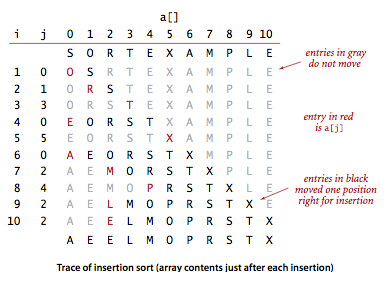### Proposition.

For randomly ordered arrays of length N with with distinct keys, insertion sort uses ~N2/4 compares and ~N2/4 exchanges on the average. The worst case is ~ N2/2 compares and ~ N2/2 exchanges and the best case is N-1 compares and 0 exchanges.

Insertion sort works well for certain types of nonrandom arrays that often arise in practice, even if they are huge. An inversion is a pair of keys that are out of order in the array. For instance, E X A M P L E has 11 inversions: E-A, X-A, X-M, X-P, X-L, X-E, M-L, M-E, P-L, P-E, and L-E. If the number of inversions in an array is less than a constant multiple of the array size, we say that the array is partially sorted.

### Proposition.

The number of exchanges used by insertion sort is equal to the number of inversions in the array, and the number of compares is at least equal to the number of inversions and at most equal to the number of inversions plus the array size.

### Property.

For randomly ordered arrays of distinct values, the running times of insertion sort and selection sort are quadratic and within a small constant factor of one another.

SortCompare.java uses the sort() methods in the classes named as command-line arguments to perform the given number of experiments (sorting arrays of the given size) and prints the ratio of the observed running times of the algorithms.

## Visualizing sorting algorithms.

We use a simple visual representation to help describe the properties of sorting algorithms. We use vertical bars, to be sorted by their heights. SelectionBars.java and InsertionBars.java produce these visualizations.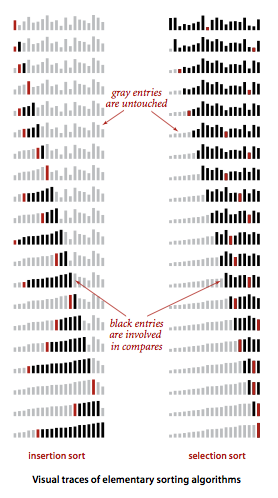## Shellsort.

Shellsort is a simple extension of insertion sort that gains speed by allowing exchanges of entries that are far apart, to produce partially sorted arrays that can be efficiently sorted, eventually by insertion sort. The idea is to rearrange the array to give it the property that taking every hth entry (starting anywhere) yields a sorted sequence. Such an array is said to be h-sorted.By h-sorting for some large values of h, we can move entries in the array long distances and thus make it easier to h-sort for smaller values of h. Using such a procedure for any increment sequence of values of h that ends in 1 will produce a sorted array: that is shellsort. Shell.java is an implementation of this method.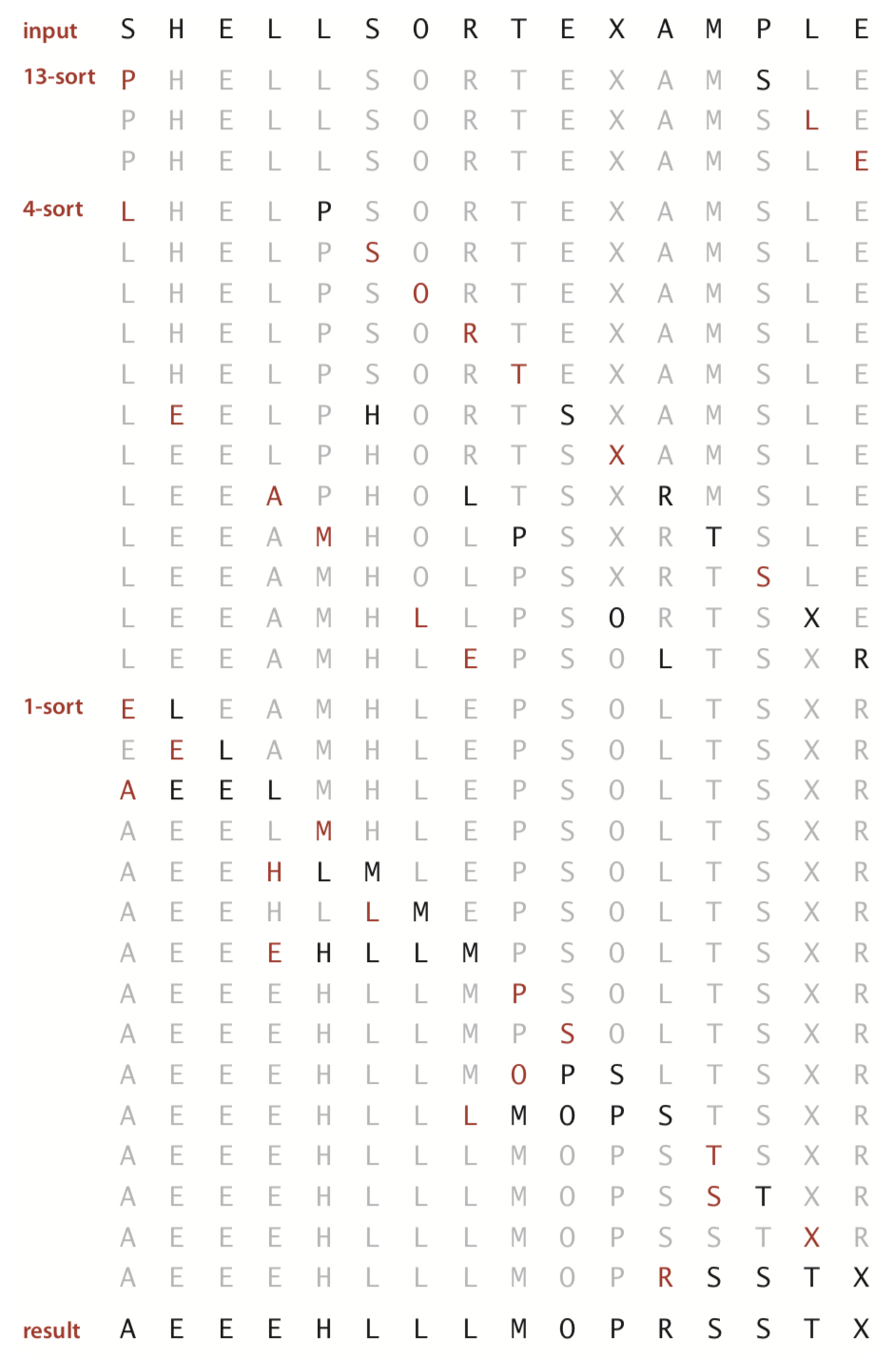ShellBars.java produces a visualization of shellsort.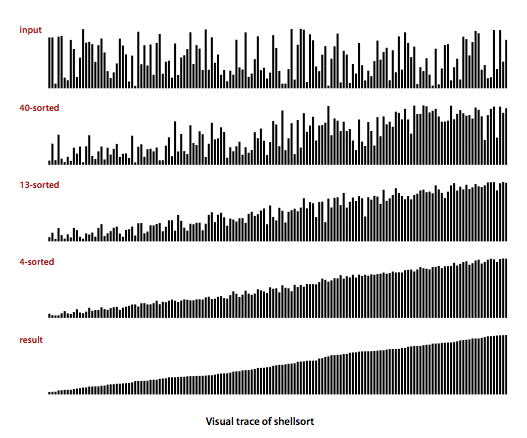### Property.

The number of compares used by shellsort with the increments 1, 4, 13, 40, 121, 364, ... is bounded by a small multiple of N times the number of increments used.

### Proposition.

The number of compares used by shellsort with the increments 1, 4, 13, 40, 121, 364, ... is O(N3/2).

#### Q + A

Q. The compiler gives a warning when I compile Insertion.java. Is ther any way to avoid this?

 Insertion.java:73: warning: [unchecked] unchecked call to compareTo(T) as a member of the raw type java.lang.Comparable return (v.compareTo(w) < 0); 

A. Yes, if you use static generics, as in InsertionPedantic.java. It leads to awkward (but warning-free) code.

#### Exercises

1. Show in the style of the example trace with selection sort, how selection sort sorts the array
 E A S Y Q U E S T I O N 

Solution.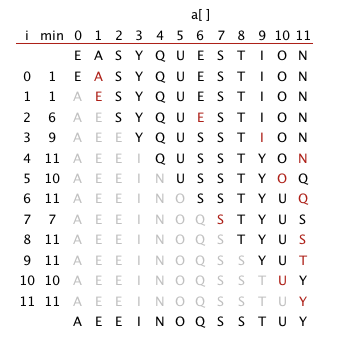2. What is the maximum number of exchanges involving any particular item during selection sort? What is the average number of exchanges involving an item?

Solution. The average number of exchanges is exactly 1 because there are exactly N exchanges and N items. The maximum number of exchanges is N, as in the following example.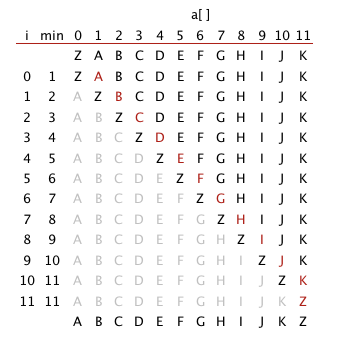3. Show in the style of the example trace with insertion sort, how insertion sort sorts the array
 E A S Y Q U E S T I O N 

Solution.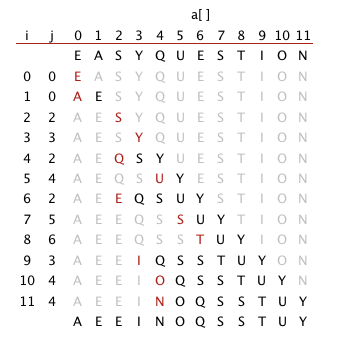4. Which method runs fastest for an array with all keys identical, selection sort or insertion sort?

Solution. Insertion sort runs in linear time when all keys are equal.

5. Suppose that we use insertion sort on a randomly ordered array where items have only one of three key values. Is the running time linear, quadratic, or something in between?

Solution. Quadratic.

6. Show in the style of the example trace with shellsort, how shellsort sort sorts the array
 E A S Y S H E L L S O R T Q U E S T I O N 

Solution.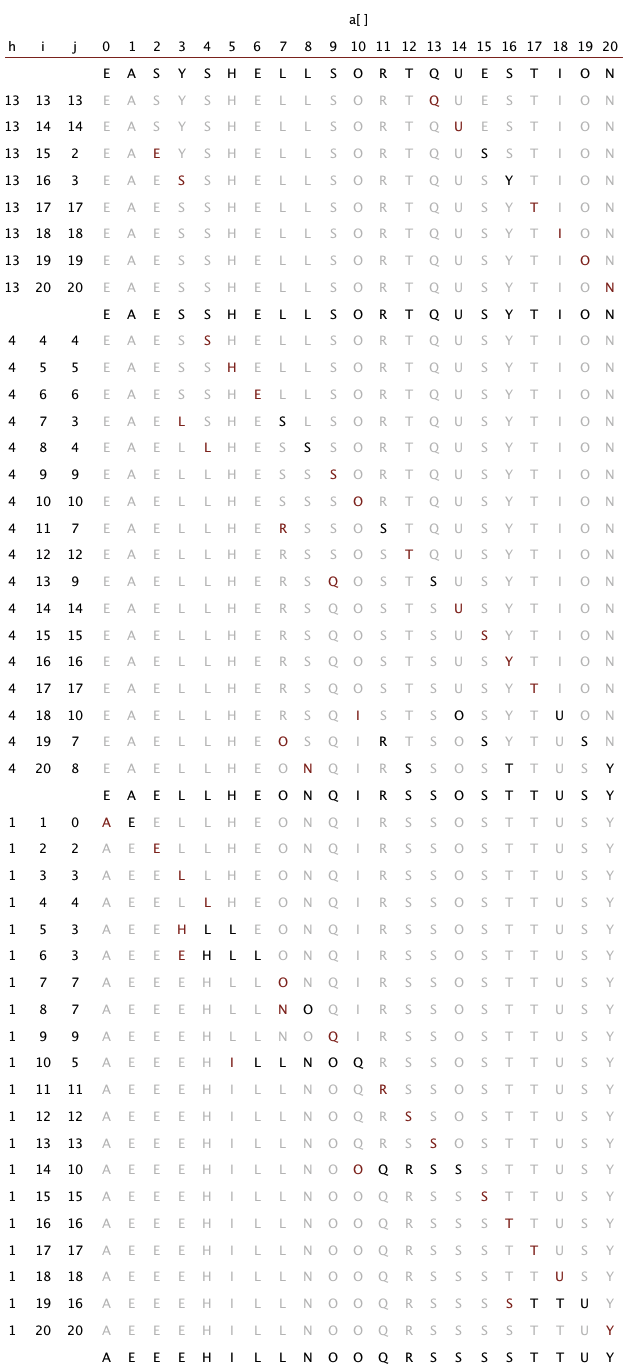7. Why not use selection sort for h-sorting in shellsort?

Solution. Insertion sort is faster on inputs that are partially-sorted.

#### Creative Problems

1. Expensive exchange. A clerk at a shipping company is charged with the task of rearranging a number of large crates in order of the time they are to be shipped out. Thus, the cost of compares is very low (just look at the labels) relative to the cost of exchanges (move the crates). The warehouse is nearly full: there is extra space sufficient to hold any one of the crates, but not two. Which sorting method should the clerk use?

Solution. Use selection sort because it minimizes the number of exchanges.

2. Visual trace. Modify your solution to the previous exercise to make Insertion.java and Selection.java produce visual traces such as those depicted in this section.

Solution. TraceInsertion.java, TraceSelection.java, and TraceShell.java.

3. Comparable transactions. Expand your implementation of Transaction.java so that it implements Comparable, such that transactions are kept in order by amount.

4. Transaction sort test client. Write a class SortTransactions.java that consists of a static method main() that reads a sequence of transactions from standard input, sorts them, and prints the result on standard output.

#### Experiments

1. Insertion sort with sentinel. Develop an implementation InsertionX.java of insertion sort that eliminates the j > 0 test in the inner loop by first putting the smallest item into position. Use SortCompare.java to evaluate the effectiveness of doing so. Note: it is often possible to avoid an index-out-of-bounds test in this way—the item that enables the test to be eliminated is known as a sentinel.

2. Insertion sort without exchanges. Develop an implementation InsertionX.java of insertion sort that moves larger items to the right one position rather than doing full exchanges. Use SortCompare.java to evaluate the effectiveness of doing so.

#### Web Exercises

1. Sorting networks. Write a program Sort3.java with three if statements (and no loops) that reads in three integers a, b, and c from the command line and prints them out in ascending order.
 if (a > b) swap a and b if (a > c) swap a and c if (b > c) swap b and c 
2. Oblivious sorting network. Convince yourself that the following code fragment rearranges the integers stored in the variables A, B, C, and D so that A <= B <= C <= D.
 if (A > B) { t = A; A = B; B = t; } if (B > C) { t = B; B = C; C = t; } if (A > B) { t = A; A = B; B = t; } if (C > D) { t = C; C = D; D = t; } if (B > C) { t = B; B = C; C = t; } if (A > B) { t = A; A = B; B = t; } if (D > E) { t = D; D = E; E = t; } if (C > D) { t = C; C = D; D = t; } if (B > C) { t = B; B = C; C = t; } if (A > B) { t = A; A = B; B = t; } 
Devise a sequence of statements that would sort 5 integers. How many if statements does your program use?
3. Optimal oblivious sorting networks. Create a program that sorts four integers using only 5 if statements, and one that sorts five integers using only 9 if statements of the type above? Oblivious sorting networks are useful for implementing sorting algorithms in hardware. How can you check that your program works for all inputs?

Answer: Sort4.java sorts 4 items using 5 compare-exchanges. Sort5.java sorts 5 items using 9 compare-exchanges.

The 0-1 principle says that you can verify the correctness of a (deterministic) sorting network by checking whether it correctly sorts an input that is a sequence of 0s and 1s. Thus, to check that Sort5.java works, you only need to test it on the 2^5 = 32 possible inputs of 0s and 1s.

4. Optimal oblivious sorting (challenging). Find an optimal sorting network for 6, 7, and 8 inputs, using 12, 16, and 19 if statements of the form in the previous problem, respectively.

Answer: Sort6.java is the solution for sorting 6 items.

5. Optimal non-oblivious sorting. Write a program that sorts 5 inputs using only 7 comparisons. Hint: First compare the first two numbers, the second two numbers, and the larger of the two groups, and label them so that a < b < d and c < d. Second, insert the remaining item e into its proper place in the chain a < b < d by first comparing against b, then either a or d depending on the outcome. Third, insert c into the proper place in the chain involving a, b, d, and e in the same manner that you inserted e (with the knowledge that c < d). This uses 3 (first step) + 2 (second step) + 2 (third step) = 7 comparisons. This method was first discovered by H. B. Demuth in 1956.
6. Stupidsort. Analyze the running time (worst case and best case), correctness, and stability of the following sorting algorithm. Scan the array from left to right until you find two consecutive items that are out-of-place. Swap them, and start over from the beginning. Repeat until the scan reaches the end of the array.
 for (int i = 1; i < N; i++) { if (less(a[i], a[i-1])) { exch(i, i-1); i = 0; } } 
Consider also the following recursive variant and analyze the worst case memory usage.
 public static void sort(Comparable[] a) { for (int i = 1; i < a.length; i++) { if (less(a[i], a[i-1])) { exch(i, i-1); sort(a); } } } 
7. Stoogesort. Analyze the running time and correctness of the following recursive sorting algorithm: if the leftmost item is larger than the rightmost item, swap them. If there are 2 or more items in the current subarray, (i) sort the initial two-thirds of the array recursively, (ii) sort the final two-thirds of the array, (iii) sort the initial two-thirds of the array again.
8. Guess-sort. Pick two indices i and j at random; if a[i] > a[j], then swap them. Repeat until the input is sorted. Analyze the expected running time of this algorithm. Hint: after each swap, the number of inversions strictly decreases. If there are m bad pairs, then the expected time to find a bad pair is Theta(n^2/m). Summing up from m =1 to n^2 yields O(N^2 log N) overall, ala coupon collector. This bound is tight: consider input 1 0 3 2 5 4 7 6 ...
9. Bogosort. Bogosort is a randomized algorithm that works by throwing the N cards up in the air, collecting them, and checking whether they wound up in increasing order. If they didn't, repeat until they do. Implement bogosort using the shuffling algorithm from Section 1.4. Estimate the running time as a function of N.
10. Slow sort. Consider the following sorting algorithm: choose two integer i and j at random. If i < j, but a[i] > a[j], swap them. Repeat until the array is in ascending order. Argue that the algorithm will eventually finish (with probability 1). How long will it takes as a function of N? Hint: How many swaps will it make in the worst case?
11. Minimum number of moves to sort an array. Given a list of N keys, a move operation consists of removing any one key from the list and appending it to the end of the list. No other operations are permitted. Design an algorithm that sorts a given list using the minimum number of moves.
12. Guess-Sort. Consider the following exchanged-based sorting algorithm: pick two random indices; if a[i] and a[j] are an inversion, swap them; repeat. Show that the expected time to sort an array of size N is at most N^2 log N. See this paper for an analysis and related sorting algorithm known as Fun-Sort.
13. Swapping an inversion. Given an array of N keys, let a[i] and a[j] be an inversion (i < j but a[i] > a[j]). Prove or disprove: swapping a[i] and a[j] strictly decreases the number of inversions.
14. Binary insertion sort. Develop an implementation BinaryInsertion.java of insertion sort that uses binary search to find the insertion point j for entry a[i] and then shifts all of the entries a[j] to a[i-1] over one position to the right. The number of compares to sort an array of length n should be ~ n lg n in the worst case. Note that the number of array accesses will still be quadratic in the worst case. Use SortCompare.java to evaluate the effectiveness of doing so.